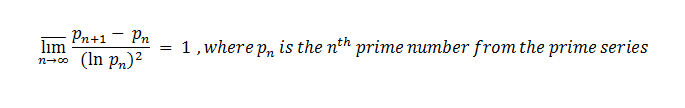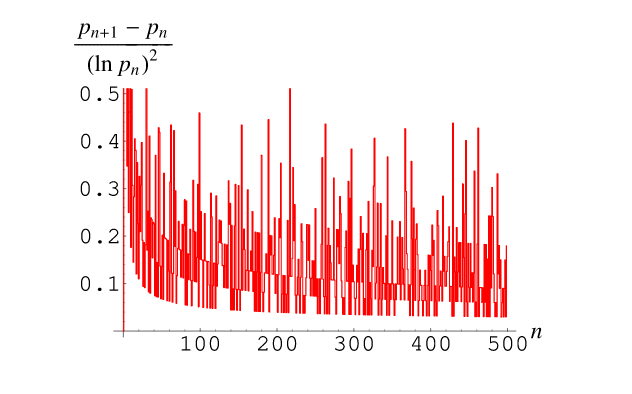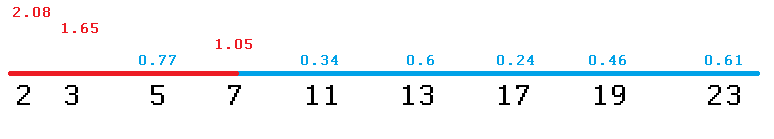×

Search anything:

# Cramer's conjecture

#### List of Mathematical Algorithms number theoryIn this article, we have explored Cramer's conjecture which is an important conjecture for prime numbers. We have developed a code to verify this conjecture.

1. Definitions
2. Algorithm
3. Time complexity
4. A way of implementation

#### 1. Definitions

A conjecture is synonymous with hypothesis and consists in having no proof yet to be verified as true of false. It starts from a real fact and it has a rule for it.

A prime number is a number that is only divisible to 1 and itself.

The first few primes are: 2, 3, 5, 7, 11, 13, 17, 19, 23, 29, 31, 37 …

Notice that 1 is not part of this series.

There is no mathematician who has not been looking at this unique series of numbers and tried to find a relation of 2 consecutive prime numbers.

We can first try to make a difference of them and check how the gap between them evolves and we can notice that except for the first 2 primes where the gap is 1 which is an odd number, the rest of the gaps are all even numbers.
Ex for the first 60 prime gaps:
1, 2, 2, 4, 2, 4, 2, 4, 6, 2, 6, 4, 2, 4, 6, 6, 2, 6, 4, 2, 6, 4, 6, 8, 4, 2, 4, 2, 4, 14, 4, 6, 2, 10, 2, 6, 6, 4, 6, 6, 2, 10, 2, 4, 2, 12, 12, 4, 2, 4, 6, 2, 10, 6, 6, 6, 2, 6, 4, 2

Do you find a pattern here ?

I've tried to find one, for example the repetition of the 4,2,4 subset, but the more series grow the more repetion diminishing and bigger even numbers start to show up.
But that doesn't help too much as we cannot predict a n-th prime number based on its predecesors, as in the factorial or Fibonacci series.

Back in 1936, Harald Cramer established an estimation for the size of the prime gaps based on a probabilistic model, essentially a heuristic one, in which the probability that a number of size x is prime is 1/log x. This is known as the Cramér random model or Cramér model of the primes.and become to be known as the Cramer's conjecture which says that the superior limit of a gap of 2 consecutive primes divided to the square root of the n-th prime number is equal with 1.#### 2. Algorithm

Since the Cramer's conjecture is a formula that is using the prime numbers, the only thing that we need to implement is to generate the prime numbers series.

If you are a beginner in programming if you want to check if a number is prime you might want to use a simple loop to check for all numbers between 2 and the square root of it !

``````    procedure isPrime(n)
loop
i <- 2..sqrt(n)
if (n mod i == 0) return false
end loop
return true
``````

This check might be time consumming and double checking. Why ? because you start checking from number 2 and continue until you reach the square root number. But in this subset there might be other numbers that are divided with other prime numbers so there is no need to check for them again !

To avoid this situation we can use an array to store the previous checked prime numbers, i.e. we only divide the given number to the prime numbers before it, this method is called memoaization.

``````procedure isPrime(array p, n)
i <- 0
while p[i] <= sqrt(n)
if (n mod p[i] == 0) return false

return true

procedure generatePrime(n)
array p
j <- 0

for i <- 3..n-1
if (isPrime(p, i))
{
p[j] <- i
j <- j + 1
}
``````

Another way is to use the sieve of Eratosthenes , the sieve of Sundaram or the sieve of Atkin

#### 3. Time complexity

Since we check all the numbers the generatePrime function will be O(N).
For the isPrime function we check only for the prime numbers before the square root of N, which depends on the size of the subset of the prime numbers which means usually O(log N)
So, generating the prime series will be O(N logN)

#### 4. A way of implementation

``````#include <iostream>
#include <math.h>

using namespace std;

bool isPrime(int *p, int n)
{
int sqrtn = sqrt(n), i=0;

while ( p[i] <= sqrtn )
if(n%p[i++] == 0) return false;

return true;
}

int & generatePrime(int n, int &size)
{
int *p, i, j;
p = new int[n];

j=0;
p[j++]=2;

for (i=3; i < n; i++)
if(isPrime(p, i)) p[j++] = i;

size = j;

return *p;
}

void checkCramerConjecture(int n)
{
int i=0, size;

int *p = &generatePrime(n,size);

while (i < size-2)
cout <<p[i]<<" "<< (p[i+1] - p[i]) / pow(log(p[i]),2) << endl,i++;
}

int main()
{
int n = 500;

checkCramerConjecture(n);

return 0;
}
``````

Output:

2 2.08137
3 1.65707
5 0.772114
7 1.05637
11 0.347832
13 0.607998
17 0.249156
19 0.461376
23 0.610294
29 0.176387
31 0.508808
37 0.306778
41 0.145026
43 0.282753
47 0.404759
53 0.380633
59 0.120291
...

The chart will be a distribution showing how the superior limit is equal with 1Why is that ? because for the first few prime numbers the Cramer's generating numbers are:which means the supremum of the series is 1.05637 meaning the least upper bound of the subset prime numbers.